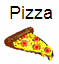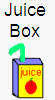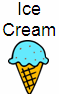Skill 8A
Picture Graphs

There are several types of visual graphs.  Here we want to determine if your student understands how to answer questions related to a picture graph.

Pretend your class had a party.  The picture graph shows the number of students eating a particular type of food. I will ask some questions about the graph.  Use the picture graph to answer the questions.1.  How many students ate pizza? 2.  How many students drank a juice box? 3.  How many students ate ice cream? 4.  Which group has more than 5? 5.  Which group has less than 5? 6.  How many more students ate pizza than ice cream? 7.  Which snack did the fewest number of students choose?